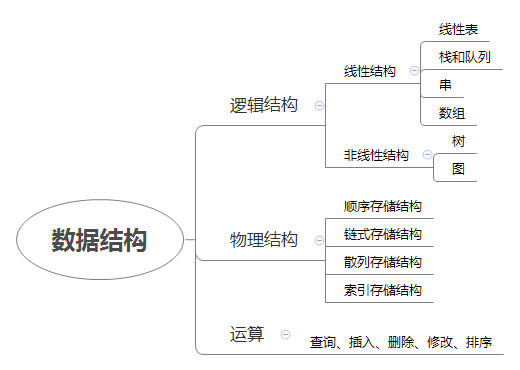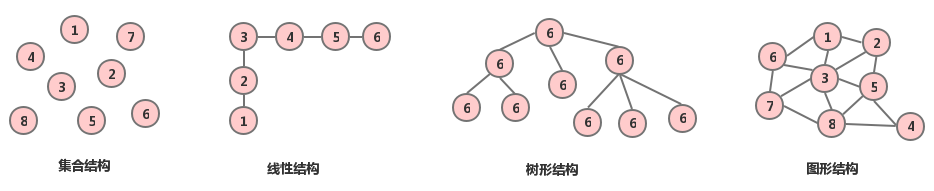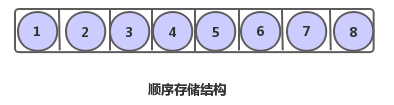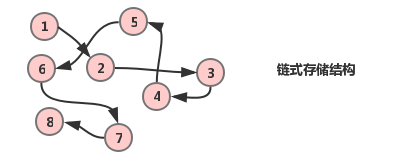11 篇文章 7 订阅

# 算法与数据结构开篇

## 你真的会数据结构吗？

• 实时排队模块，在内存中实现即可，无序用数据库

• 数组虽然可以满足一定需求，但是需要考虑溢出问题，以及新增和删除后的数据移动，显然不是很方便

## 为什么你的程序比别人的慢？(算法问题)

//i j k 分别代表公鸡 母鸡 雏鸡的数量
//20、34、300 为100元最多能买公鸡、母鸡、小鸡的数量
for (int i = 0; i < 20; i++) {
for (int j = 0; j < 34; j++) {
for (int k = 0; k < 300; k = k + 3) { //k = k + 3 是为了满足小鸡实际存在的要求
if (5*i + 3*j +  k/3 == 100 && i + j + k == 100) {
cout << "公鸡 母鸡 雏鸡 数量分别为：" << i <<", "<< j <<", "<< k << endl;
}
}
}
}


for (int i = 0; i < 20; i++) {
for (int j = 0; j < 34; j++) {

k_temp = 100 - i - j;

if (k_temp % 3 == 0)
k = k_temp;

if (5*i + 3*j +  k/3 == 100 && i + j + k == 100) {
cout << "公鸡 母鸡 雏鸡 数量分别为：" << i <<", "<< j <<", "<< k << endl;
}
}
}


int i, j, k, t;
for (int t = 0; t <= 25/7; t++) {
i = 4 * t;
j = 25 - 7 * t;
k = 75 + 3 * t;
cout << "公鸡 母鸡 雏鸡 数量分别为：" << i <<", "<< j <<", "<< k << endl;
}


//根据花钱的总数为100列式
5x + 3y + (100 - x - y)/3 = 100

//对上式进行化简
y = 25 -(7/4)x
= 25 - 2x + x/4

//设 x/4 = t，原式子可变为
y = 25 - 7t

//可以得到三个不等式
x = 4t >= 0
y = 25 - 7t >= 0
z = 75 + 3t >= 0

//解得
t >= 0
t <= 25/7


#include<ctime>
clock_t startTime,endTime;
startTime = clock();

......测试代码

endTime = clock();
cout << "The run time is: " << (double) (endTime - startTime) / CLOCKS_PER_SEC << "s" << endl;


The run time is: 0.114s
The run time is: 0.03s
The run time is: 0.026s


##为什么要一起学习数据结构和算法？

## 数据结构的基本概念和术语

• 数据：描述客观事物的数字和符号，是能够被计算机识别且进行处理的的符号集合

• 整型和实数型数据是可以直接进行数值计算的

• 文字、图像、图形、声音、视频等多媒体信息则可以通过合适的编码保存处理

例如：文本通过字符编码处理、声音通过储存波形 (WAV) 或在波形分解后存储其振幅特性然后压缩 (MP3)

• 数据元素：组成数据且有意义的的基本单位 (个体)

• 例如：猫和狗都是动物群体中的一个数据元素
• 数据项：组成数据元素具有特定意义的最小不可分割单位

• 作为人这个类群中具体的个体，一个人而言，其所拥有的手、脚、眼、鼻，亦或者姓名、年龄、身高、等都属于数据项

• 数据对象：性质相同的数据元素的集合，是数据的子集

• 例如：人类中一些人的生日、身高相同

## 逻辑结构和物理结构(存储结构)### 逻辑结构

• （每一个数据元素看做一个节点，元素之间的逻辑关系用连线表示，如果关系有方向则连线带箭头）### 物理结构(存储结构)

• 也就是说：元素一个一个有序的排好队，各自占据一定的空间，例如定义一个含有6个浮点型数据的数组：然后内存中的一块大小为6个浮点型数据大小空间就会被计算机所开辟，然后数据存入时，依次顺序摆入• 因为数据元素位置不确定，所以需要通过指针指向到元素的存储地址，从而确定不同数据元素之间的位置

• 举例：200人同时在一个阶梯教室上课，同学们坐的位置是没有关系的，老师点名签到的时候，你只需要关注你学号前一位的同学有没有被点到，点到后，你就知道下一个该你了• 你别慌，我这就来解释了，它的原理就是，将一个节点的关键字key作为自变量，通过一个确定的函数运算f(key)，其函数值作为节点的存储地址，将节点存入到指定的位置上，查找的时候，被搜索的关键字会再次通过f(key)函数计算地址，然后读取对应数据

• 我们后面会专篇讲解这个内容，现在做一个简单的了解即可

## 算法的特征

• 输入：算法具有零个或者多个输入（零个的情况例如打印输出字符串，或者算法自身已经给定了初始条件）

• 输出：算法具有一个或者多个输出，用来反映算法对输入数据加工后的结果

• 有穷性：算法必须在执行有限个步骤后终止

• “有限” 的定义不是绝对的，而是实际应用中合理的可接受的
• 确定性： 算法的每一步骤都具有确定的含义，不会出现二义性

• 也就是说，唯一的输入只有唯一的输出
• 可行性：算法的每一步都是可行的，通过有限步骤可以实现

## 算法的设计要求

• 正确性：合理的数据输入下，最终可以输出能解决问题需求的正确答案

• 对正确的理解：
• 无语法错误
• 输入合法和非法的数据均可以得到正确答案
• 输入刁难的数据依旧可以输出满足需要的答案
• 可读性：算法便于阅读和理解

• 算法应该层次分明，易读易懂，方便二次调试和修改

• 复杂一些的算法，变量的命名尽量恰当一些，用阿里的开发手册中的一句话就是说：“正确的英文拼写和语法可以让阅读者易与理解避免歧义”“为了达到代码自解释的目标，任何自定义编程元素在命名时，使用尽量完整的单词组合来表达其意”

• 健壮性：当数据不合理的时候，算法也能对各种情况作出处理，而不是报出异常，或者输出错误的答案

• 高效性：尽量满足时间效率高，存储率低的需求

## 函数的渐进增长

n = 16623
n = 28946
n = 3101269
n = 1024332030
n = 100204303200300

n = 1的时候 算法 A 和 B 的效率一致，但是从n = 2的时候开始算法 A 的效率已经大于算法 B 了，n值变大后，算法 A 相比 B 变的更加快速

• -所以在n值没有限定的情况下，只要在超过某数值N后，函数值就一直大于另一个函数，那么我们称函数是渐进增长的

函数的渐近增长：给定两个函数f(n)和g(n)，如果存在一个整数N，使得对于所有的n > N，f(n)总是比g(n)大，那么，我们说f(n)的渐近增长快于g(n)

• 接着我们分别用 AC 、BD进行对照，你会发现其实后面的加数对算法的影响几乎是忽略不计的，所以我们一般选择忽略这些加法常数

n = 18611
n = 2101524
n = 3123039
n = 102630310100
n = 1002063000310010000

• 最高次项的系数对数据走向的影响不大

• 最高项的指数大的函数，随着n的增长，结果也会快速增长

• 判断算法效率的时候，应主要关注最高次项，其他次要项或者常数项常常可以忽略

## 算法的时间复杂度

• T(n) = O(f(n)) 解释：随着n增大算法执行时间的增长率和f(n)的增长率相同，同时也考察输入值大小趋于无穷的情况，也称作算法的渐进时间复杂度

• 在这个过程中，不考虑函数的低阶项、常数项和最高项系数，

### 常见的时间复杂度

O(1) < O(logn) < O(n) < O(nlogn) < O(n²) < O(n³) < O(2^n) < O(n!) < O(n^n)

### 结尾：12-0622万+08-27107
07-1038
09-2513万+
06-167万+
02-042万+
01-161496
08-12698
07-211092
03-2659
11-047119
08-3062
02-099万+
03-3167
03-271万+
11-29156
10-14740
02-24190点击重新获取扫码支付余额充值